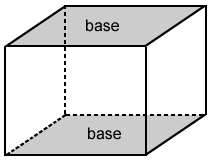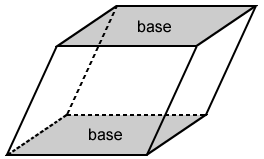# Rectangular prism

A rectangular prism is a prism with rectangular bases. The lateral faces of a rectangular prism are parallelograms. Below are a few examples of rectangular prisms.

A rectangular prism is a shape that is commonly seen in everyday life. A box, an eraser, and an aquarium are all examples of real life objects that can be in the shape of a rectangular prism.

## Characteristics of a rectangular prism

A rectangular prism is enclosed by six faces consisting of two rectangular bases and four lateral faces in the shape of a parallelogram. A rectangular prism has 12 edges and 8 vertices. The 12 edges of a rectangular prism are in 3 groups of parallel lines. The parallel edges are equal in length.

The opposite faces are parallel and congruent to each other. Any cross section that is parallel to a face of a rectangular prism has the shape of a rectangle or parallelogram and is congruent to the face.

Two rectangular cross sections for the rectangular prism are shown in green above. They are congruent to the two rectangular bases of the prism since they are formed by cross sections that are in planes parallel to the bases. This is true for any cross section parallel to the faces of a rectangular prism.

## Rectangular prism classifications

Rectangular prisms can be classified based on how their bases and lateral faces intersect or meet. A right rectangular prism has bases that are perpendicular to its lateral faces, meaning that they meet at right angles. A rectangular prism that doesn't meet these conditions is instead an oblique rectangular prism. A right rectangular prism is also called a cuboid.

Right rectangular prism Oblique rectangular prismThere are also rectangular prisms that have specific names based on their characteristics:

• A cube is a right rectangular prism with all square faces.
• A right square prism is a right rectangular prism with square bases.

## Volume of a rectangular prism

The volume, V, of a rectangular prism is

V = l·w·h

where l is the length of the base, w is the width of the base, and h is the height of the prism. For a right rectangular prism, the height is its vertical edge. For an oblique rectangular prism, the height is the perpendicular distance from any point on one base to the other base.